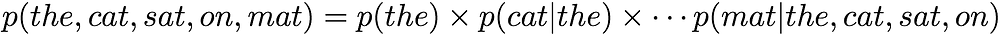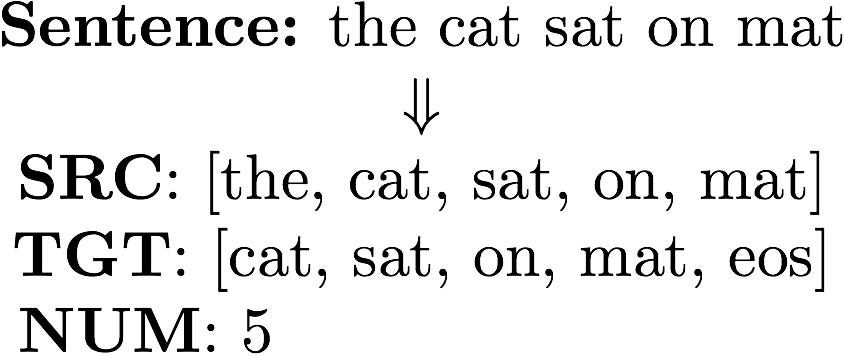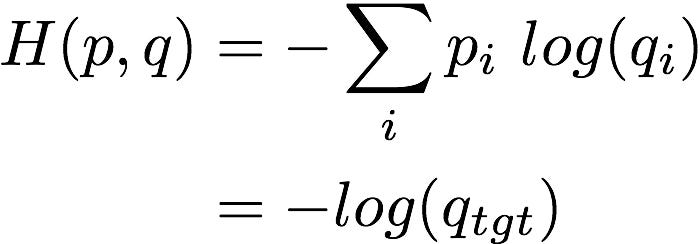Building a Neural Language Model

Step by Step using Tensorflow Eager

I am privileged this Fall to teach a course on fundamentals of Deep Learning at IIT Delhi. While preparing to teach RNN, I was amazed how easy tf.eager makes it to build models with RNN. The ease is primarily as you can execute your code right there and see how your model works. Thus, you can quickly create a prototype of your model using tf.eager and Jupyter notebook.

In this post, we will leverage the official tutorial on eager for Language Modeling as a base, and learn how to build a Neural Language Model, step by step. As always, the excruciatingly detailed notebooks and helper code is available on github. So, let us begin!

Language Model (LM)

A language model (LM) can predict a word given previous words in a sentence. More concretely, LM can generate a conditional probability distribution, where `w_t` represents a word at position `t`. In the remainder of the post we will use position and time step interchangeably.

For example, for a sentence: `The cat sat on mat`, LM can compute the following:

Why would this be useful? We can use LM to compute how likely a sentence is by decomposing joint probability:Computing joint probability for a sentence “the cat sat on mat” using a LM

One should think of LM as a statistical model, which consumes words seen in a sequence, and generates a probability distribution for the next word.

Let us get started by looking at some code!

Enable Eager

Enable eager. This should be done right at the start of your code execution!

Feeding Words

Inputs to a LM are words. A statistical model cannot directly work with text. Thus the first step is to convert a string word to an integer. Once we have a unique index for a word, we further convert the index to a word vector of dimension `d`. Think of word vector as a feature representation for a word. It is important to understand that this feature representation differs from standard machine learning feature representation in atleast two ways. First, the feature is not provided but learnt. Second, an individual feature does not carry any meaning as such: features are looked together. Thus these features are also known as distributed representations.

In Tensorflow, we can build a simple Embedding model which would take as input a Tensor of word indexes and return the corresponding word vectors. Here `V` represents the size of the vocabulary:Embedding Model: returns word vectors for an input tensor of word indexes

Note, how Embedding model can take input of any shape:

RNN Cell

We now have the ability to feed vectors for each time step. Now let us say we see two words and want to predict the third word in a sentence. We need a mechanism that can summarize all the words seen so far, and use the summary to generate a probability distribution for the next word.

Recurrent Neural Network (RNN) does precisely that: It maintains a lossy summary of the inputs seen so far! More precisely, RNN computes a hidden state `h_t` given an input `x_t` and the previous hidden state at last time step:

Here `f` is what differentiates a RNN Cell. There are quite a few varieties: BasicRNNCell, GRUCell, LSTMCell to name a few. We are not going to go into further details, but there is a well written post on LSTM in case you are interested. In this post, we are going to treat RNNCell as a black box which consumes a fresh input, a previously computed state, and returns back a new state. We are also going to remember one more thing: LSTM and GRU is good at capturing long term dependencies: that is if input at say time step 1 effects input at time step 10. BasicRNN is not good at remembering long term dependencies!

Batched Inputs

In Tensorflow, we always feed inputs as a batch. This means that we give a bunch of training examples together. Thus if batch size is 32, we feed 32 sentences at one go. At time step 1, we feed the first word from the group of 32 sentences together:

In the above example, word_vectors would be of shape `(2, 3, 128)`. Remember though that we want to feed inputs ordered by time. We can reshape the data and create a list using tf.unstack. We want to create a list according to time which is the second dimension, thus we specify `axis=1`. Thus we get 3 members of size `(2, 128)`:Convert batch_size x T x d to a list of batch_size x d with T members.

Now, we are ready to feed inputs to a RNN cell:RNN Cell computation. cell can be replaced with LSTM/GRU without changing any other code!

When we begin computation, we need to specify an initial state. We start with a zero state (Line 2). It is important to note that we only talked about hidden state `h_t` till now, but we have two vectors being computed: `output` and `state`. For a BasicRNNCell output and state are identical. For LSTM and GRU they have a different meaning. All we need to understand for now, is that `state` and `output` is used by LSTM and GRU to do its magic of learning long term dependencies. We will use `state` to pass it to the next time step, and `output` to make predictions at the current time step.

Now, we know enough to build an RNN Model, which can take a batch of word_vectors and return outputs for each time step…

Data pipeline

Let us now think about how we will read a raw data file, and feed it for training a LM. Let us start with a popular dataset: PTB Dataset

Here are some interesting things to note about this dataset. We already have a unknown token <unk>. The words are tokenized, lower cased and separated by space. Finally, all numbers are replaced by N. Let us now count how many unique words are in our dataset:

We have 9999 words in our vocabulary. We are going to add one more word to our vocabulary now: end of sequence (<eos>). This signifies that the sentence has added. Next, we write the list of words to a file, which we will use later to convert a word to integer:

Peek at the newly created vocab file, and see if the words make sense. We wrote <unk> as the first word and <eos> as the second word for ease of demonstration. It is important to understand that ordering of words does not matter here, as long as we assign a unique index to each word!Vocab file created when words are written in order of frequency (highest first)

Now, let us create a data pipeline which would directly read a text file and create Tensors for our Language Model. This concept is extremely powerful as it separates out data from model! We can replace data file (which we will for say our validation dataset) and we can reuse the data pipeline! We will use tf.data to do so. If you are unfamiliar with using tf.data you can refer to my earlier post. We basically want to change an input sentence as follows:Transform a single sentence to SRC, TGT and num of words in a sentence

Now, since we want integers, we will lookup an integer for each individual word in SRC and TGT. Also, we will finally create a batch of say 32 sentences at one go:

We will now see how a batch of data looks. This is where we can see how eager behaves as native python! We get three components: source words, target words and number of words. Each component is a created using a batch of 32 sentences:

RNN Model (Revisited)

We will now see how a batch of data created using our data pipeline, can be fed to RNN. Let us revisit how we can create vectors from sentences:

We can now feed the word vectors to our RNN Model. We get a list of 48 outputs (corresponding to 48 time steps). Each output has a batch of 32 outputs:

One problem with our current RNN implementation is that it processes even past the sentence length. For example, length of sentence 0 is 24, but since longest sentence in first batch is of length 48. It returns outputs even past length 24:

This is bad, as we don’t want to get any output beyond the sentence length. We can either multiply outputs by zeros, or directly use tf.nn.static_rnn which takes a sequence length as input:

Language Model (Code)

We have not talked about converting RNN output which is of `h` dimensions to predict a probability distribution over `V` words. Well, all we need is a Dense layer. We now get `V=10000` logits (corresponding to each word in our vocabulary) at each time step

Loss function

We will use cross entropy (CE) to compute the loss between predictions made by our model, and the ground truth. Cross entropy measures the distance between two probability distributions. In our case, the true probability distribution has only one element as 1 (the correct word), and rest all entries are zero. Thus it can be directly simplified as follows where `tgt` is the true label word:Cross entropy when one label is true in the labels or p

Now, before we jump into how CE is computed in tensorflow. Let us get an intuition of what would be CE for a model that makes random predictions. Well that is easy to compute. We get 9.2103:

Okay, let us see what does our untrained model return. We are not doing any better than a random model. That is fine, as we have not even trained our model!

The next thing we need to be careful about is how is loss computed for the padded words. More concretely, we do not want to add any loss for sentence 0 past length 24! Let us check what is loss:

We will need to fix this! We will multiply the loss we computed using a mask of 1’s and 0’s, such that all losses past sentence length are zeroed out:

Finally let us wrap our loss function. We will need to be careful to compute average loss by dividing it by total number of words in the batch:

Once we define a loss function, tf.eager makes it extremely easy to compute gradients:

Training Loop

Writing training loop using eager is elegant. It reads as if you are iterating over a Python container!